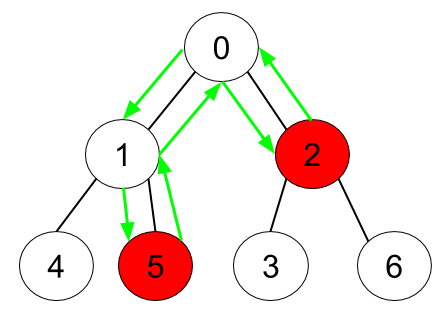Formatted question description: https://leetcode.ca/all/1443.html

# 1443. Minimum Time to Collect All Apples in a Tree (Medium)

Given an undirected tree consisting of n vertices numbered from 0 to n-1, which has some apples in their vertices. You spend 1 second to walk over one edge of the tree. Return the minimum time in seconds you have to spend in order to collect all apples in the tree starting at vertex 0 and coming back to this vertex.

The edges of the undirected tree are given in the array edges, where edges[i] = [fromi, toi] means that exists an edge connecting the vertices fromi and toi. Additionally, there is a boolean array hasApple, where hasApple[i] = true means that vertex i has an apple, otherwise, it does not have any apple.

Example 1:Input: n = 7, edges = [[0,1],[0,2],[1,4],[1,5],[2,3],[2,6]], hasApple = [false,false,true,false,true,true,false]
Output: 8
Explanation: The figure above represents the given tree where red vertices have an apple. One optimal path to collect all apples is shown by the green arrows.


Example 2:Input: n = 7, edges = [[0,1],[0,2],[1,4],[1,5],[2,3],[2,6]], hasApple = [false,false,true,false,false,true,false]
Output: 6
Explanation: The figure above represents the given tree where red vertices have an apple. One optimal path to collect all apples is shown by the green arrows.


Example 3:

Input: n = 7, edges = [[0,1],[0,2],[1,4],[1,5],[2,3],[2,6]], hasApple = [false,false,false,false,false,false,false]
Output: 0


Constraints:

• 1 <= n <= 10^5
• edges.length == n-1
• edges[i].length == 2
• 0 <= fromi, toi <= n-1
• fromi < toi
• hasApple.length == n

Related Topics:
Tree, Depth-first Search

## Solution 1. Post-order Traversal

// OJ: https://leetcode.com/problems/minimum-time-to-collect-all-apples-in-a-tree/

// Time: O(N)
// Space: O(N)
class Solution {
vector<vector<int>> G;
vector<bool> seen;
int dfs(int u, vector<bool> &hasApple) {
seen[u] = true;
int ans = 0;
for (auto v : G[u]) {
if (seen[v]) continue;
ans += dfs(v, hasApple);
}
if (!ans && !hasApple[u]) return 0;
return u == 0 ? ans : ans + 1;
}
public:
int minTime(int n, vector<vector<int>>& E, vector<bool>& hasApple) {
seen.assign(n, false);
G.assign(n, {});
for (auto & e : E) G[e].push_back(e), G[e].push_back(e);
return 2 * dfs(0, hasApple);
}
};


Java

class Solution {
public int minTime(int n, int[][] edges, List<Boolean> hasApple) {
Map<Integer, Set<Integer>> adjacentMap = new HashMap<Integer, Set<Integer>>();
for (int[] edge : edges) {
int node0 = edge, node1 = edge;
Set<Integer> set0 = adjacentMap.getOrDefault(node0, new HashSet<Integer>());
Set<Integer> set1 = adjacentMap.getOrDefault(node1, new HashSet<Integer>());
}
Map<Integer, Integer> parentMap = new HashMap<Integer, Integer>();
Map<Integer, Set<Integer>> childrenMap = new HashMap<Integer, Set<Integer>>();
parentMap.put(0, -1);
boolean[] visited = new boolean[n];
visited = true;
int[] distances = new int[n];
Arrays.fill(distances, Integer.MAX_VALUE);
distances = 0;
queue.offer(0);
while (!queue.isEmpty()) {
int node = queue.poll();
int distance = distances[node];
Set<Integer> childrenSet = childrenMap.getOrDefault(node, new HashSet<Integer>());
for (int nextNode : adjacentSet) {
if (!visited[nextNode]) {
parentMap.put(nextNode, node);
visited[nextNode] = true;
distances[nextNode] = distance + 1;
queue.offer(nextNode);
}
}
childrenMap.put(node, childrenSet);
}
int minTime = 0;
boolean[] bottomUpVisited = new boolean[n];
for (int i = 0; i < n; i++) {
if (hasApple.get(i)) {
bottomUpVisited[i] = true;
bottomUpQueue.offer(i);
}
}
while (!bottomUpQueue.isEmpty()) {
int node = bottomUpQueue.poll();
int parent = parentMap.get(node);
if (parent >= 0) {
minTime += 2;
if (!bottomUpVisited[parent]) {
bottomUpVisited[parent] = true;
bottomUpQueue.offer(parent);
}
}
}
return minTime;
}
}